## Abstract

The effect of transpiration on magnetohydrodynamic stagnation-point flow of a Carreau nanofluid toward a stretching/shrinking sheet in the presence of thermophoresis and Brownian motion was investigated numerically. The transformed governing partial differential equations are solved using Runge–Kutta coupled with shooting technique. The effect of pertinent parameters on velocity, temperature and concentration profiles along with the friction factor, local Nusselt and Sherwood numbers is presented graphically and through tables. It is observed that, increasing values of the thermophoresis parameter enhances the heat and mass transfer rate, whereas the Weissenberg number enlarges the momentum boundary layer thickness along with the heat and mass transfer rate. A good agreement of the present results has been observed by comparing with the published results.

## Keywords

MHD; Carreau fluid; Thermophoresis; Brownian motion; Stagnation-point flow

## 1. Introduction

The problem of convective heat and mass transfer past a permeable stretching/shrinking sheet plays a vital role in manufacturing industries in design of reliable equipments. The study of fluid flow through a stretching sheet is a wide area of research, and it has gained the attention of many researchers due to its enormous industrial and engineering applications such as extrusion of plastic and rubber sheets, glass blowing, cooling/drying of papers and textiles, hot rolling polymer processing industries, glass fiber and textile production, petroleum production, crystal growing, wire drawing, fiber spinning, and the cooling of nuclear reactors. In view of these wide varieties of applications Crane  has pioneered the work on steady two-dimensional flow over a permeable stretching sheet. Sandeep and Sulochana  studied the influence of chemical reaction on MHD mixed convective micropolar fluid flow through a stretching/shrinking surface in the presence of non-uniform heat generation/absorption and observed the dual solutions. Singh and Chamkha  observed the dual solutions for viscous fluid flow and heat transfer toward a linearly shrinking plate with second order slip. Nadeem et al.  discussed the suction/injection effects on MHD Casson nanofluid flow through a nonlinearly shrinking surface in the presence of convective boundary conditions. This study was further extended by many authors such as Mansur et al. , Qasim and Noreen , Robert et al. , Lok and Pop , Mahapatra and Samir Kumar , Sharma et al. , Makinde et al. , Raju et al. , Bhattacharyya , Prakash et al. , Yacob et al. , and Wahiduzzaman et al.  to investigate several aspects such as suction/blowing, viscous dissipation, thermal radiation, Buoyancy effects, chemical reaction, and heat generation over a stretching/shrinking surface.

In the recent years, the problem of stagnation point flow has received tremendous research interest. Stagnation-point flow is seen in every type of flow whenever the fluid impinges on a solid object. Then, the fluid velocity reduces to zero and the fluid pressure and heat mass transfer rates are highest at the stagnation point area. The studies of stagnation-point flow toward a stretching or shrinking sheet are classic in fluid mechanics because of its enormous practical applications in industry and engineering, such as cooling of nuclear reactors and cooling of electronic devices by fans, in the design of radial diffusers and thrust bearings, drag reduction and many hydrodynamic processes. In view of aforesaid applications Wang  studied the stagnation point flow through a shrinking sheet and observed that due to increase in boundary layer thickness the heat transfer rate decreases with the rate of shrinking. The effect of induced magnetic field on MHD stagnation point flow and heat transfer of an viscous incompressible fluid through a stretching surface is studied by Ali et al.  and they compared their results in the absence of magnetic field parameters with previously derived results and the results are found to be good. Akbar et al.  analyzed the influence of Brownian motion, thermophoresis and radiation effects on steady two-dimensional stagnation point flow of nanofluid past a stretching cylinder in the presence of convective boundary conditions. Rushi Kumar et al.  studied the effect of chemical reaction on MHD flow through a vertical cone with variable electrical conductivity. A lot of work on stagnation-point flow is done by the researchers , , , , , , , , , , , ,  and .

Moreover, shear stress is directly proportional to shear rate of both Newtonian and non-Newtonian fluids. Carreau fluid is a type of Newtonian fluid. The study of peristaltic flow of a Carreau fluid attracted the attention of many researchers because of its wide applications in the field of science and technology viz. Physiology, treatment of diagnostic diseases, neurological treatment, cancer treatment. The effect of magnetic field on a Carreau (Newtonian) fluid has been used for treatment of gastronomic pathologies, hypertension, cancer tumor treatment, hyperthermia, blood reduction during surgeries, etc. Although many researchers such as Raju et al.  and , Akbar et al. , Riaz et al. , Jasmine Benazir et al.  and Nadeem et al.  addressed the non-Newtonian fluid through different channels, in all the aforesaid investigations, a less work has been available on the flow of Carreau fluids past a stretching/shrinking sheet. Very recently, the researchers , ,  and  investigated the heat and mass transfer in magnetohydrodynamic flows by considering the Buongiorno’s model.

In this study, we investigated the influence of transpiration on the flow of a Carreau nanofluid near a stagnation-point toward a stretching/shrinking sheet in the presence of Brownian motion and thermophoresis effects. The governing partial differential equations are transformed into nonlinear ordinary differential equations by using appropriate similarity transformations and then solved numerically. The influence of non-dimensional governing parameters namely magnetic field parameter, power-law index, Weissenberg number, Brownian motion, thermophoresis and stretching/shrinking parameter on velocity, temperature and concentration profiles along with friction factor, local Nusselt and Sherwood numbers is discussed and presented through graphs and tables for both suction and injection cases and obtained the dual solutions.

## 2. Mathematical formulation

Consider a steady two dimensional stagnation point flow of an incompressible Carreau nanofluid over a wall coinciding with plane y = 0, and the flow is being confined to ${\textstyle y>0}$. A magnetic field of strength ${\textstyle B_{0}}$ is applied along x-direction as displayed in Fig. 1. Induced magnetic field is neglected in this study. Thermophoresis and Brownian motion effects are taken into account. The flow is generated due to the linear stretching. Extra stress tensor for Carreau fluid is ,

 ${\overline {\tau }}_{ij}={\eta }_{0}\left[1+{\frac {\left(n-1\right)}{2}}{\left(\Gamma {\overline {\overset {\cdot }{\gamma }}}\right)}^{2}\right]{\overline {\overset {\cdot }{\gamma }}}_{ij}$
(1)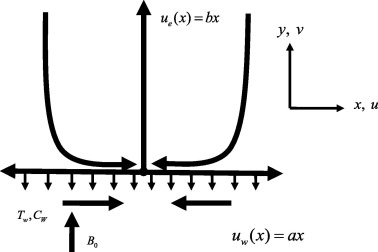Figure 1. Physical model of the problem.

In which ${\textstyle {\overline {\tau }}_{ij}}$ is the extra stress tensor, ${\textstyle {\eta }_{0}}$ is the zero shear rate viscosity, ${\textstyle \Gamma }$ is the time constant, n   is power law index and ${\textstyle {\overline {\overset {\cdot }{\gamma }}}_{ij}}$ is defined as

 ${\overline {\overset {\cdot }{\gamma }}}={\sqrt {{\frac {1}{2}}{\sum }_{i}{\sum }_{j}{\overline {\overset {\cdot }{\gamma }}}_{ij}{\overline {\overset {\cdot }{\gamma }}}_{ji}}}=$${\sqrt {\frac {1}{2}}}\prod {\mbox{.}}$
(2)

Here ${\textstyle \prod }$ is the second invariant strain tensor. The physical model of the problem is displayed in Fig. 1.

Flow equation for Carreau fluid model after applying the boundary layer approximations can be defined as follows:

 ${\frac {\partial u}{\partial x}}+{\frac {\partial v}{\partial y}}=$$0{\mbox{,}}$
(3)

 $u{\frac {\partial u}{\partial x}}+v{\frac {\partial u}{\partial y}}=$${\nu }_{f}{\frac {{\partial }^{2}u}{\partial y^{2}}}+{\nu }_{f}{\frac {3(n-1){\Gamma }^{2}}{2}}{\left({\frac {\partial u}{\partial y}}\right)}^{2}{\frac {{\partial }^{2}u}{\partial y^{2}}}+$${\frac {\sigma {\beta }_{0}^{2}}{{\rho }_{f}}}(u_{e}-u)+u_{e}{\frac {\partial u_{e}}{\partial x}}{\mbox{,}}$
(4)

 $u{\frac {\partial T}{\partial x}}+v{\frac {\partial T}{\partial y}}=$${\frac {k_{f}}{{\rho }_{f}c_{p}}}{\frac {{\partial }^{2}T}{\partial x^{2}}}+$$\tau \left(D_{B}{\frac {\partial C}{\partial y}}{\frac {\partial T}{\partial y}}+\right.$$\left.{\frac {D_{T}}{T_{\infty }}}{\left({\frac {\partial T}{\partial y}}\right)}^{2}\right){\mbox{,}}$
(5)

 $u{\frac {\partial C}{\partial x}}+v{\frac {\partial C}{\partial y}}=$$D_{B}{\frac {{\partial }^{2}C}{\partial y^{2}}}+{\frac {D_{T}}{T_{\infty }}}\left({\frac {{\partial }^{2}T}{\partial y^{2}}}\right){\mbox{,}}$
(6)

Here u and v are velocity components along x and y   directions, respectively, where ${\textstyle {\nu }_{f}}$ is kinematic velocity, ${\textstyle \sigma }$ is the electrical conductivity, ${\textstyle {\rho }_{f}}$ is the density of the base fluid and ${\textstyle D_{B}\quad {\mbox{and}}\quad D_{T}}$ are the Brownian motion and thermophoretic diffusion coefficients. It is noticed that for power law index ${\textstyle \left(n=1\right)}$ our problem reduced to the case of Newtonian fluid while for ${\textstyle n>1}$ phenomena remains for non-Newtonian fluid. The corresponding boundary conditions are

 ${\begin{array}{c}u=u_{w}(x)=ax{\mbox{,}}\quad v=v_{w}(x){\mbox{,}}\quad T=T_{1}{\mbox{,}}\quad C=C_{1}\quad {\mbox{at}}\quad y=0\\u\rightarrow u_{e}(x)=bx{\mbox{,}}\quad v\rightarrow 0{\mbox{,}}\quad T\rightarrow T_{2}{\mbox{,}}\quad C\rightarrow C_{2}\quad {\mbox{as}}\quad y\rightarrow \infty \end{array}}$
(7)

In which ${\textstyle b>0}$ is constant, we assume that ${\textstyle u_{w}(x)=ax}$ and ${\textstyle u_{e}(x)=bx}$ are the velocities near and away from the wall respectively. Introducing the following similarity transformations

 $\eta ={\sqrt {{\frac {b}{\nu }}_{f}}}y{\mbox{,}}\quad \psi =$${\sqrt {b{\nu }_{f}}}xf(\eta ){\mbox{,}}\quad \theta (\eta )=$${\frac {T-T_{\infty }}{T_{w}-T_{\infty }}}{\mbox{,}}\quad \phi (\eta )=$${\frac {C-C_{\infty }}{C_{W}-C_{\infty }}}$
(8)

where η   is the similarity variable and ${\textstyle \psi }$ is the stream function defined in the usual notation as ${\textstyle u=\partial \psi /\partial y}$ and ${\textstyle v=-\partial \psi /\partial x}$.

Which identically satisfy the equation of continuity defined in Eq. (3). By using aforesaid similarity transformation defined in Eq. (7) on Eqs. (2), (3), (4), (5) and (6), we get the following:

 $f^{'''}-{\left(f^{'}\right)}^{2}+{ff}^{''}+1+{\frac {3(n-1){We}^{2}}{2}}f^{'''}{\left(f^{''}\right)}^{2}+$$M^{2}(1-f^{'})=0{\mbox{,}}$
(9)

 $Pr\quad {\theta }^{''}+f{\theta }^{''}+Nb\quad {\theta }^{'}{\phi }^{'}+$$Nt{\left({\theta }^{'}\right)}^{2}=0{\mbox{,}}$
(10)

 ${\phi }^{''}+{\frac {Nt}{Nb}}{\theta }^{''}+Scf{\phi }^{'}=$$0{\mbox{,}}$
(11)

The boundary conditions (7) reduce to

 ${\begin{array}{l}f=s{\mbox{,}}\quad f^{'}=\lambda {\mbox{,}}\quad \theta =1{\mbox{,}}\quad \phi =1\quad {\mbox{at}}\quad \eta =0\\f\rightarrow 0{\mbox{,}}f^{'}\rightarrow 1{\mbox{,}}\quad \theta \rightarrow 0{\mbox{,}}\quad \phi \rightarrow \quad 0\quad {\mbox{as}}\quad \eta \rightarrow \infty \end{array}}$
(12)

where ${\textstyle s=-v_{w}/{\left(av\right)}^{1/2}{\mbox{,}}\quad s>0(i.e.v_{w}<0)}$ corresponding to suction and ${\textstyle s<0(i.e.v_{w}>0)}$ corresponding to blowing case. Here ${\textstyle \lambda =a/b}$ is the stretching/shrinking parameter, ${\textstyle {We}^{2}={\frac {b^{3}x^{2}{\Gamma }^{2}}{\nu }}}$ is the Weissenberg number, ${\textstyle M^{2}={\frac {\sigma B_{0}^{2}}{\rho b}}}$ is the magnetic parameter, ${\textstyle Pr={\frac {k}{\mu c_{p}}}}$ is the Prandtl number, ${\textstyle Nb={\frac {\tau D_{B}(C_{w}-C_{\infty })}{\nu }}}$ is the Brownian motion parameter, ${\textstyle N_{t}={\frac {\tau D_{T}(T_{w}-T_{\infty })}{\nu T_{\infty }}}}$ is the Thermophoresis parameter, and ${\textstyle Sc={\frac {\nu }{D_{B}}}}$ is the Schmidt number. After using boundary layer approximations wall shear stress ${\textstyle {\tau }_{w}}$ is given by

 ${\tau }_{w}={\frac {\partial u}{\partial x}}+{\frac {(n-1){\Gamma }^{2}}{2}}{\left({\frac {\partial u}{\partial y}}\right)}^{3}$
(13)

The coefficient of skin friction is defined as

 $c_{f}={\frac {{\tau }_{w}}{\rho u_{w}^{2}}}$
(14)

In dimensionless form skin friction is defined as

 ${\sqrt {Re}}c_{f}={\left[f^{''}(\eta )+{\frac {(n-1){We}^{2}}{2}}{\left(f^{''}(\eta )\right)}^{3}\right]}_{\eta =0}$
(15)

The local Nusselt and Sherwood numbers are given by

 ${Re}_{x}^{-1/2}{Nu}_{x}=-{\theta }^{'}(0){\mbox{,}}$
(16)
 ${Re}_{x}^{-1/2}{Sh}_{x}=-{\phi }^{'}(0){\mbox{,}}$
(17)

## 3. Results and discussion

The nonlinear ordinary differential Eqs. (9), (10) and (11) with respect to the boundary conditions (12) are solved numerically using Runge–Kutta based shooting technique (Sandeep and Sulochana ). In order to get the clear insight into the problem, the results obtained show the influence of the non-dimensional governing parameters namely Magnetic field parameter M, power-law index n, thermophoresis parameter Nt, Brownian motion parameter Nb  , stretching/shrinking parameter ${\textstyle \lambda }$, Weissenberg number We on velocity, temperature and concentration profiles of the flow. In the present study, for numerical computation we have considered the non-dimensional parameter values such as We = 0.3; M = 1; Nb = 0.1; Nt = 0.1; Pr = .7; Sc = 0.6; ${\textstyle \lambda =2}$. These values are kept as common in the entire study except the varied values as shown in respective figures and tables. In the present study we considered two cases such as suction and injection and obtained dual solutions by keeping S value S > 0 and S < 0 for suction and injection cases respectively.

Figure 2, Figure 3, Figure 4 and Figure 9 depict the influence of the non-dimensional parameters such as magnetic field M, power-law index n, Weissenberg number We   and stretching/shrinking parameter ${\textstyle \lambda }$ on velocity profiles of the flow. It is evident from Fig. 2 that rising values of the magnetic field parameter M depreciate the velocity profiles for both suction and injection cases. This is due to the fact that the effect of horizontal magnetic field on electrically conducting fluid creates a drag force and develops the body force known as Lorentz force. This force reduces the motion of the fluid; hence, it causes to reduce in the velocity field. From Figure 3 and Figure 4 it is noticed that increasing values of the power-law index parameter n and Weissenberg number We enhances the velocity profiles for both the cases. Physically, rising values of the power-law index increase the nonlinearity of the sheet, and this helps to reduce the resistive force. In Fig. 9 it is observed that increasing values of ${\textstyle \lambda }$ enhances the velocity profiles for both cases. Physically, rising values of the stretching parameter enhance the stretching velocity of the flow. This leads to increase the momentum boundary layer thickness.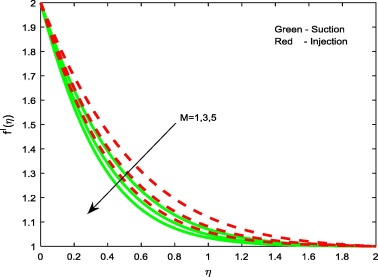Figure 2. Velocity profiles for different values of M.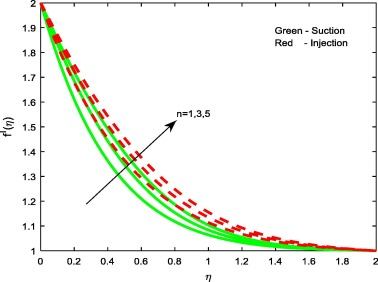Figure 3. Velocity profiles for different values of n.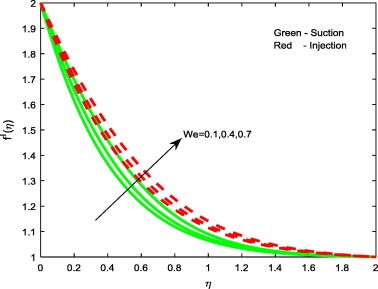Figure 4. Velocity profiles for different values of We.

Figure 5, Figure 7 and Figure 10 display the influence of the non-dimensional parameters such as thermophoresis parameter Nt, Brownian motion parameter Nb   and stretching/shrinking parameters ${\textstyle \lambda }$ on temperature profiles of the flow. From Figure 5 and Figure 7 it is evident that increasing values of Nt and Nb enhances the temperature profiles for both the cases. This is due to the fact that, different nanoparticles have different values of Nt and Nb. This leads to different heat transfer rate. These two particles can be used to control the heat transfer rate in a nanofluid. As a result, boundary layer thickness of the thermal field increases with enhancement in the values of Nt and Nb  . But we have noticed opposite trend in case of stretching/shrinking parameter. That is rise in ${\textstyle \lambda }$ declines the temperature profiles for both the cases. This leads to enhance the heat transfer rate.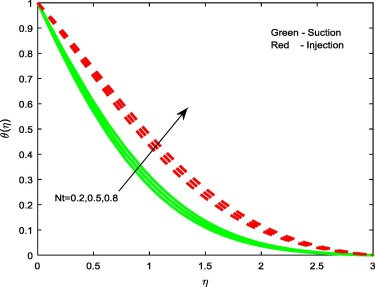Figure 5. Temperature profiles for different values of Nt.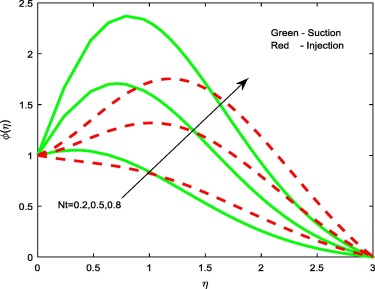Figure 6. Concentration profiles for different values of Nt.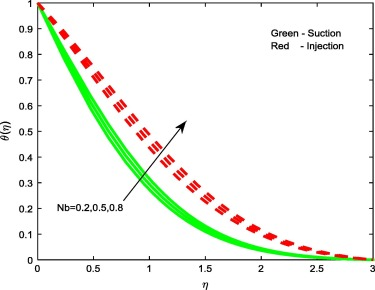Figure 7. Temperature profiles for different values of Nb.

Figure 6, Figure 8 and Figure 11 reveal the effect of thermophoresis parameter Nt, Brownian motion parameter Nb   and Stretching/shrinking parameter ${\textstyle \lambda }$ on concentration profiles of the flow. Brownian motion and thermophoresis parameters have an opposite effect on concentration profiles (see Figure 6 and Figure 8). Fig. 6 portrays that the enhancement in the value of Nt improves the concentration profiles for both the cases, whereas in case of Brownian motion parameter and stretching/shrinking parameter, increase in Nb   and ${\textstyle \lambda }$ depresses concentration profiles for both the cases. The reason behind this is Brownian motion helps to heat the fluid in the boundary layer and simultaneously exacerbates particle deposition away from the fluid regime or onto the surface; thereby, it is seen reduction in the concentration profiles.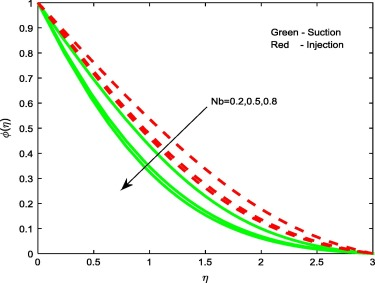Figure 8. Concentration profiles for different values of Nb.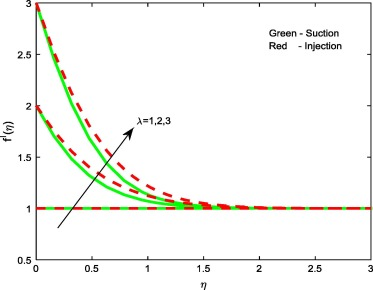Figure 9. Velocity profiles for different values of ${\textstyle \lambda }$.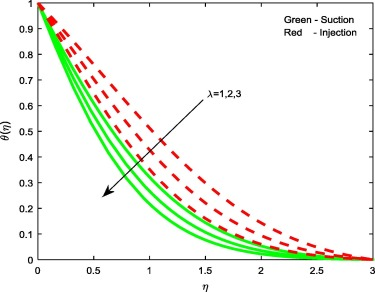Figure 10. Temperature profiles for different values of ${\textstyle \lambda }$.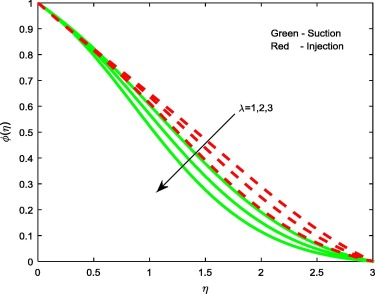Figure 11. Concentration profiles for different values of ${\textstyle \lambda }$.

Table 1 and Table 2 depict the effects of non-dimensional governing parameters on friction factor, local Nusselt and Sherwood numbers for both suction and injection cases. It is evident from the tables that rise in the magnetic field and thermophoresis parameters depreciates the heat and mass transfer rate. As we discussed above, the thermophoresis and Brownian motion values are not uniform for all nanoparticles. This leads to decline the heat and mass transfer rate. Increasing values of non-Newtonian parameter and stretching parameter enhances the heat and mass transfer rate but reduces the skin friction coefficient. A similar type of result has been observed with the increase in Weissenberg number. Physically, rising values of the Weissenberg number boost-up the thermal conductivity of the flow, and hence the heat transfer rate. Table 3 depicts the validation of the present study by comparing with the existed studies. We found a good agreement of the present results. This proves the validity of the present results along with the numerical technique used in the present study.

Table 1. Variation in ${\textstyle f^{''}(0)}$, ${\textstyle -{\theta }^{'}(0)}$ and ${\textstyle -{\phi }^{'}(0)}$ at different non-dimensional parameters for suction case.
M n We Nt Nb ${\textstyle \lambda }$ ${\textstyle f^{''}(0)}$ ${\textstyle -{\theta }^{'}}$ (0) ${\textstyle -{\phi }^{'}}$ (0)
1 −2.525906 1.013520 0.445605
3 −3.003430 1.005620 0.445503
5 −3.428428 0.999722 0.445533
1 −2.410951 1.008874 0.445806
3 −2.740310 1.021423 0.445569
5 −2.964644 1.028777 0.445835
0.1 −2.426350 1.009514 0.445770
0.4 −2.593440 1.016104 0.445561
0.7 −2.815233 1.023965 0.445684
0.2 −2.525086 0.962819 −0.284024
0.5 −2.525087 0.901708 −1.843874
0.8 −2.525087 0.844223 −3.096175
0.2 −2.525087 0.951278 0.640987
0.5 −2.525087 0.857436 0.838972
0.8 −2.525087 0.770151 0.887667
1 0.000000 0.860143 0.308584
2 −2.525086 0.984021 0.309574
3 −6.100597 1.112523 0.319893

Table 2. Variation in ${\textstyle f^{''}(0)}$, ${\textstyle -{\theta }^{'}(0)}$ and ${\textstyle -{\phi }^{'}(0)}$ at different non-dimensional parameters for injection case.
M n We Nt Nb ${\textstyle \lambda }$ ${\textstyle f^{''}(0)}$ ${\textstyle -{\theta }^{'}}$ (0) ${\textstyle -{\phi }^{'}}$ (0)
1 −1.968908 0.615412 0.530400
3 −2.447425 0.606141 0.530317
5 −2.871317 0.599465 0.530344
1 −1.884604 0.612845 0.530420
2 −2.146035 0.620488 0.530376
3 −2.344675 0.625674 0.530468
0.1 −1.895195 0.613175 0.530414
0.4 −2.022650 0.617008 0.530374
0.7 −2.211498 0.622251 0.530399
0.2 −1.967600 0.550167 0.210043
0.5 −1.967600 0.509256 −0.157505
0.8 −1.967600 0.471057 −0.341803
0.2 −1.967600 0.545343 0.480295
0.5 −1.967600 0.490702 0.541782
0.8 −1.967600 0.439984 0.556700
1 0.000000 0.446399 0.372719
2 −1.967600 0.564433 0.376997
3 −4.902551 0.685096 0.384657

Table 3. Comparison of the present results for skin friction coefficient when ${\textstyle M=Pr=Sc=Nt=Nb=We=0{\mbox{,}}\quad n=1}$ .
${\textstyle \lambda }$ Akbar et al.  Mahapatra and Samir Kumar  Present results
0 1.2326 1.2326 1.232612
0.1 1.1466 1.1466 1.146610
0.2 1.0511 1.0511 1.051101
0.3 0.7133 0.7133 0.713311

## 4. Conclusions

This study presents a numerical solution for analyzing the heat and mass transfer in the Carreau nanofluid near the stagnation-point toward a permeable stretching/shrinking sheet in the presence of Brownian motion and thermophoresis effects. Conclusions of the present study are as follows:

• Rise in non-Newtonian parameter effectively enhances the heat and mass transfer rate.
• Magnetic field parameter has tendency to control the flow field.
• Dual solutions exist only for certain range of suction/injection parameter.
• Momentum, thermal and concentration boundary layers are highly significant in injection case while compared with the suction case.
• Weissenberg number has tendency to enlarge the momentum boundary layer thickness along with the heat and mass transfer rate.

### Document informationPublished on 12/04/17

Licence: Other

### Document Score0

Views 43
Recommendations 0